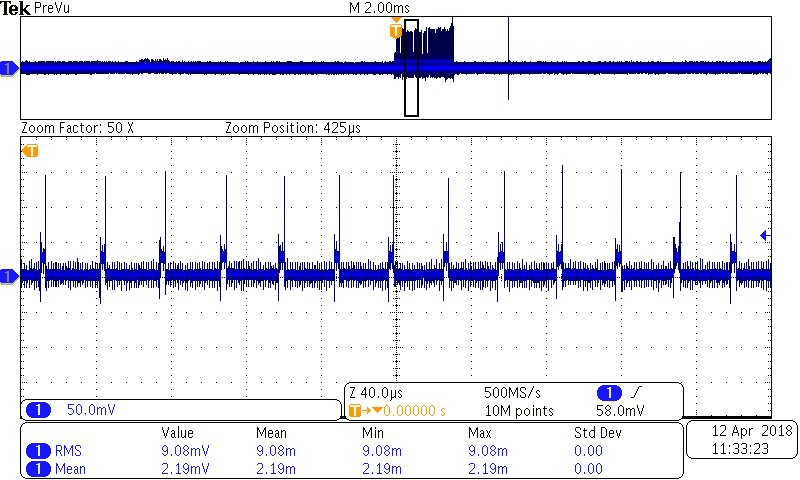If you have a related question, please click the "Ask a related question" button in the top right corner. The newly created question will be automatically linked to this question.

# UCC28910: Changing output voltage

Part Number: UCC28910

Using the UCC28910FBEVM-526 eval kit and would like to change the output voltage to 6.5V.

I thought it could be done by just changing the values of R9=105kOhm and R8=20kOhm, however it appears that the device enters a shutdown mode whenever I apply a load to the secondary of the transformer.

• Hello Michael,

The reason why the device shutsdown is because there is a under voltage shutdown current on VS of 75uA and the enable current threshold is 210 μA.
I would suggest checking the current to the VS pin.

Kind Regards,
Sonal
• Sonal,

I am measuring 594uA peaks into VS at 120VAC in. Is peak current the correct measurement?

Using the equations given for calculating RS1 and RS2 with a NPA = 4.71 and NPS = 16.5:
RS1 = ((80VAC*sqrt(2))/(4.71*210uA) = 114kOhm => 105kOhm
RS2 = (4.05V * 105000Ohm * 4.71) / (((6.5V +0.7V)*16.5)-(4.05V*4.71)) = 20.08 kOhm => 20kOhm

Is there something I am missing?

Thanks,
Mick
• Michael,

1. IVSL_run is the current you see at the VS pin during the ON tim e of the controller. This value should be used fort he calculation of RS1 and RS2.
2. The current @ VS pin should typically be 215uA (260uA max), you are dumping too much current into it.

Regards,
Sonal
• This is a capture across a 100Ohm resistor into the VS pin. Looks like ~20uA which would explain the issue.The question is, if I am using 210uA in the calculations shown earlier, how do I correct this?

Regards,

Mick

• Hello Mick,

I noticed two things:

• The NPA=5.17 and NPS=16.5 in the EVM how is your ratio different.
• The voltage drop across the diode is different from the one in the EVM have you changed the diode?

I would suggest to put the original values in the divider and check for 5Vout regulation to make sure if other components have been damaged.

Sonal

• Sonal,

The NPA and NPS values were taken off of this datasheet https://www.mouser.com/datasheet/2/445/750313739-1222373.pdf . I have not changed the transformer. I have swapped out the secondary side diode to https://www.mouser.com/ds/2/258/mcc_ss12-ss110hsma-1179975.pdf (SS110). We have confirmed that when reverting back to the original values of 100k and 30k that the EVM is in regulation with this diode. I will however revert back to the original diode to test.

Regards,

Mick

• Hello Sonal,

The transformer datasheet from the EVM references a 4.71 turns ratio for NPA. There may have been a chance in the EVM or datasheet which is causing the mismatch.

• Enos/Mick,

I don't think of any reason that might trigger the controller shutdown given you changed RS1/2. If the controller is operating with original values I would suggest kepping RS1 the same as original value and changing RS2 to 19kOhm. Using a diffirent value of RS1 might be changing the internal gain of the controller.
Doing this you would have also increased the turn ON voltage by 80*sqrt(2)*6.5/5= 147 Vrms. What is the input voltage of your operation have you tried to increase the Vin?

Also worth while checking the voltage on every pin of the controller and making sure that they are within the spec.

Regards,
Sonal
• Sonal,

Thanks for the help!

Going back to the original diode on the EVM allows for regulation at 6.5V. Based on the datasheet, it call out for a reverse voltage with at least 30% margin (100V was used) and a current rating 2-5 times rated maximum draw (1A was used for a 500mA max load). Are there other considerations that we need to account for when selecting the secondary diode?

Thanks,
Mick
• I would also make sure that it is a fast recovery diode.
Good to know that your problem is solved.

Sonal